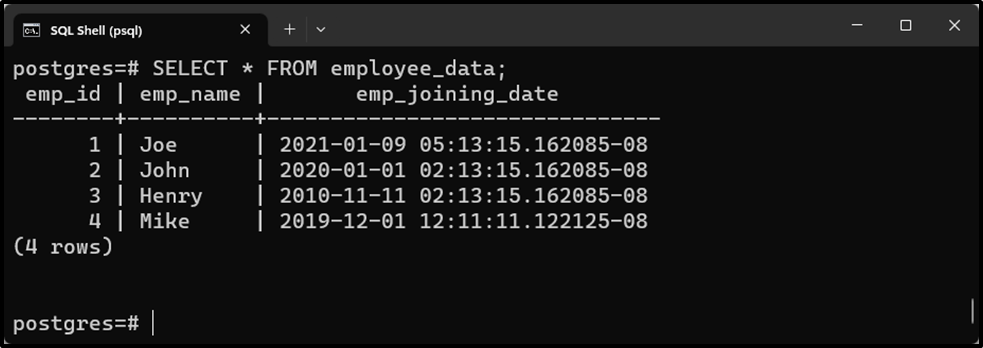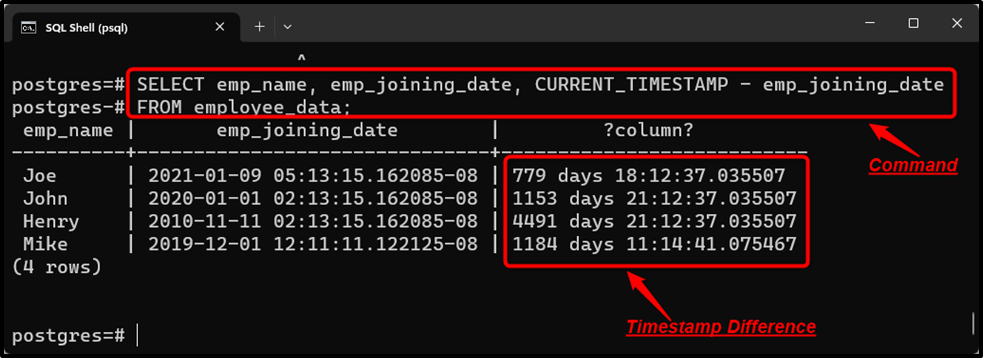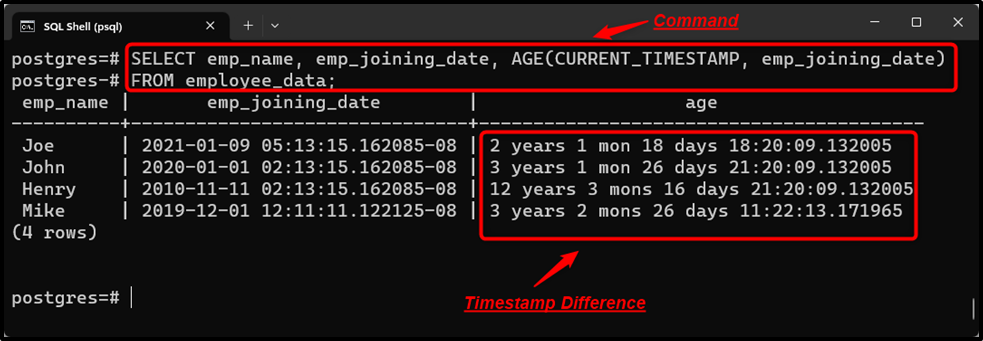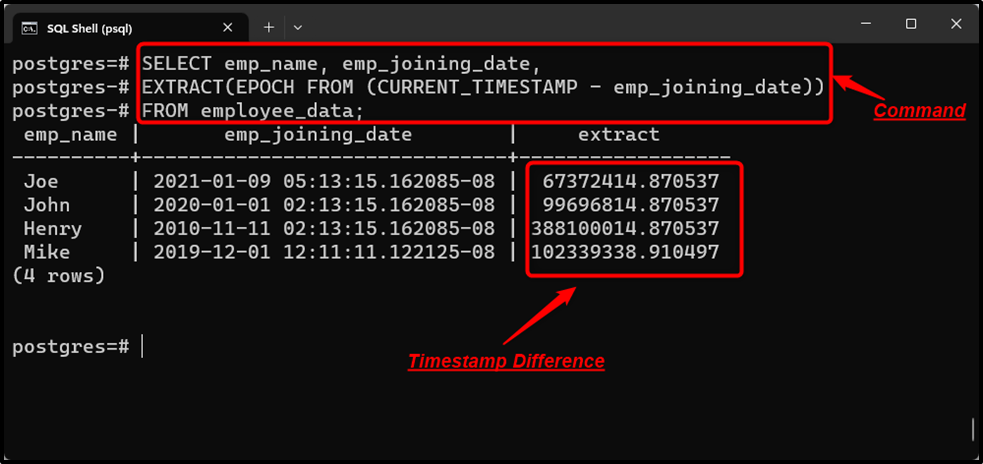##### How to Find Difference Between Two TIMESTAMPS in PostgreSQL

In PostgreSQL, the AGE() function, the minus operator “-”, and the EXTRACT() function is used to get the difference between two timestamps. The “-” operator and AGE() function return the timestamp difference as an interval. While to get the TIMESTAMPS difference in seconds, the EXTRACT() function is executed along with the EPOCH.

This post demonstrates how to find timestamp differences in Postgres using the AGE() function, the minus “-” operator, and the EXTRACT() function.

How to Find the TIMESTAMPS Difference in Postgres Using Minus Operator?

Use the below syntax to find the timestamp difference via the “-” operator:

`TIMESTAMP 'TIMESTAMP_2' - TIMESTAMP 'TIMESTAMP_1';`

The return type of the resultant value will be “INTERVAL”.

Example: Finding Timestamp Difference Using “-” Operator

A sample table named “employee_data” has already been created in the database with the following data:Suppose we have to subtract the “emp_joining_date” from the CURRENT_TIMESTAMP to get the TIMESTAMP difference as an interval:

```SELECT emp_name, emp_joining_date, CURRENT_TIMESTAMP - emp_joining_date
FROM employee_data;```The “-” operator successfully retrieves the timestamp difference in INTERVAL.

How to Find the TIMESTAMP Difference in Postgres Using AGE() Function?

A timestamp difference as an INTERVAL can be obtained by passing the given timestamps to the AGE() function:

`AGE('timestamp_1', 'timestamp_2');`

Example: Finding Timestamp Difference Using AGE() Function

In this example, the AGE() function is utilized to fetch the difference between the current timestamp and the employee’s joining_date:

```SELECT emp_name, emp_joining_date, AGE(CURRENT_TIMESTAMP, emp_joining_date)
FROM employee_data;```The AGE() function retrieves the timestamp difference as an interval.

How to Find the TIMESTAMPS Difference in Postgres Using EXTRACT() Function?

In Postgres, the EXTRACT() function is used with the collaboration of the EPOCH to get the timestamp difference in seconds:

`EXTRACT(EPOCH FROM (timestamp_1 - timestamp_2));`

Let’s put this concept into practice for a profound understanding.

Example: Finding Timestamp Difference in Seconds

The below-given statement illustrates how to utilize the EXTRACT() function to calculate the timestamp difference in seconds:

```SELECT emp_name, emp_joining_date,
EXTRACT(EPOCH FROM (CURRENT_TIMESTAMP, emp_joining_date))
FROM employee_data;```The output snippet proves that the “EXTRACT()” function succeeds in finding the timestamp difference in seconds.

Conclusion

In PostgreSQL, the minus operator “-”, the EXTRACT(), and the AGE() functions are used to find the difference between the given timestamps. The “-” operator and AGE() function retrieves the timestamp difference as an interval. While the EXTRACT() function is used with the collaboration of the EPOCH and it returns the timestamp difference in seconds. This post presented a thorough overview of finding the timestamp difference using the “-” operator, the AGE() function, and the EXTRACT() function.